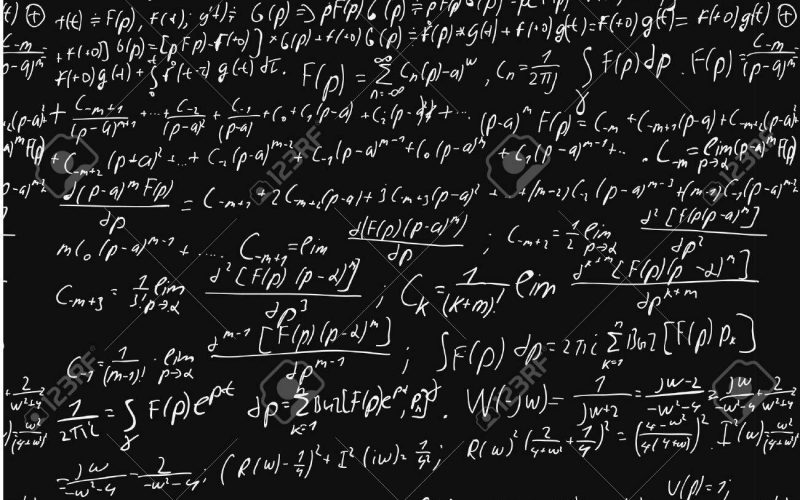# THE ASPECTS WHICH THE PROBABILITY CALCULATIONS SHOW

This post is also available in: German, Turkish

Let us examine the magnificent table that presents the names of God with the probability calculations, in order to show the greatness of the miracle 19 and how it is that such a miracle could be the result of coincidence.

Since the probabity is 1/19 for any word in Basmalah to be repeated is a multiple of 19, for four words it is 1/194.

If we take into account this probability, along with the probability of the repetition of the word “Witness” (Shahid), which replaces the word “name,” to be 19 too, then our probability is 1/195 (Because it is important to find exactly the number 19 rather than anything that is multiple of 19 here, its probability is lower).

Both in the Basmalah and in the table of God’s attributes, the coefficients of the 4 words are 1+142+3+6= 152 (19×8). The probability of the coefficient number being a multiple of 19 is 1/19. If we add this to the previous number, then we have 1/196.

We have to calculate the numerical value of God’s attributes separately on the right side, because these numbers are not only multiples of 19, but they also exactly correspond to the same numbers on the left side. The biggest mathematical value of any of God’s names is 2698. There are 2698 whole numbers up to 2698. We can show our set like this: (1, 2, 3, 4, ……, 2696, 2697, 2698). The probability of finding exactly the number that we want from this set of numbers is 1/2698. If we repeat this operation four times then the probability of finding the numbers we want is 1/26984. The probability we have found up to now is 1/196 x 1/26984:

Here is the result… Read it if you can!
1/2.492.811.198.929.640.000.000

This unreadable number is sufficient in itself to show how impossible it is to form even a single 19 table by chance. Since this number represents the probability of forming a single table like that, who can come up with the assertion that there is not a 19 code in the Quran, which Quran itself says that it will dispel suspicion? Even the probability of a single table shows the Quran’s miracle and how unchangeable it is.

There are other aspects that would serve to lessen the probability. For example, the total of the four values on both sides is 5776 (5776 = 42 x 192). In this table, a system with 19, with symmetry of four numbers on each side, can be seen. If we take the numbers 4 and 19 shown by this table, and if we multiplied by the number 2 to the second power, which shows the two sides, it would be equal to the total of all the numbers. It is seen that by doing so we could add a few digits to the denominator. But, because it would be difficult to show why we added this result to the probability, and because of the complexity of the calculation, we did not add this to the final result. Apart from this, we mentioned that 19 was the 8th prime number. On the two sides of the table we examined eight names of God, and the total of the coefficients on both sides was 152 (19×8). But we did not take these aspects, which could lessen the probability, into consideration.